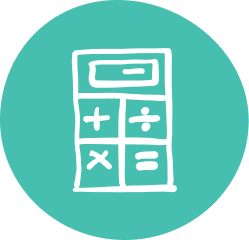### Quality Grade 3 Math Tutoring & Help

Along with math skills, 3rd Grade math tutoring will help your child gain school confidence and learn focus and study skills that will be used for years to come.

In Grade 3, your child is learning more advanced math concepts that build upon the foundation of Grades 1 and 2. Math doesn’t always click, and some children can’t keep up with the classroom pace. As students in Grade 3 start to explore more abstract math principles, ensure your child is on track for math success with Oxford Learning®’s Grade 3 math help programs!

Math can become more complicated for students as new concepts are introduced. Math is cumulative, building one skill on the previous; if a concept is missed, students can fall behind. The Grade 3 math tutors at Oxford Learning® are trained to teach thinking and learning strategies to help your child make sense of math and build their math comprehension.

### Grade 3 Math Tutoring Subjects

##### Number Sense/Calculation
• Use currencies of up to 10 dollars
• Add, subtract, multiply, and divide intermediate number combination
##### Measurement
• Understand and measure distance in kilometres
• Calculate mass in kilograms and litres
• Measure area and perimeter on grid paper
##### Geometry/Spatial Sense
• Identify right angles
• Identify and categorize a variety of geometric shapes
• Understand and identify quadrilateral shapes
##### Algebra/Patterning
• Graph different patterns and sequences
##### Data Management/Probability
• Organize discrete data into graphs
• Learn the concept of mode